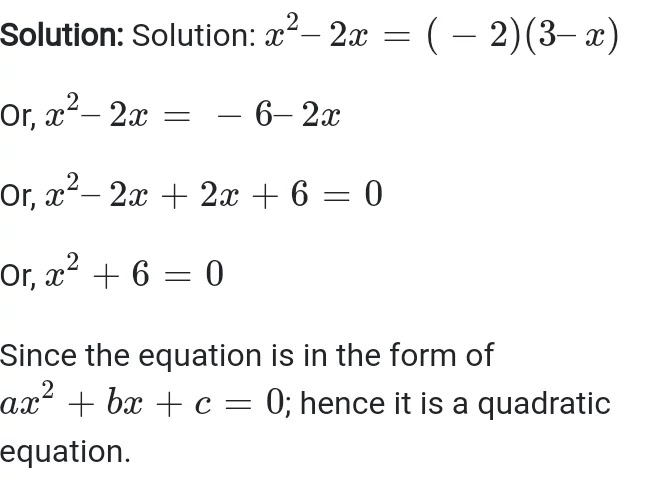NCERT Solutions Class 10 Maths Chapter 4 Quadratic Equations – Here are all the Class 10 Maths Chapter 4 Quadratic Equations NCERT Solutions. This solution contains questions, answers, images, step by step explanation of the complete Chapter 4 titled Quadratic Equations in Class 10. If you are a student of Class 10 who is using NCERT Textbook to study Maths, then you must come across Chapter 4 Quadratic Equations. After you have studied lesson, you must be looking for answers to its textbook questions. Here you can get complete NCERT Solutions for Class 10 Maths Chapter 4 Quadratic Equations in one place.

Class 10 Maths Ex. 4.1

# Exercise 4.1 Class 10 Maths Question 1 Solution

Ex. 4.1 Class 10 Question 1.

Check whether the following are quadratic equations:

(i) (x + 1)2 = 2(x – 3)

(ii) x2 – 2x = (–2) (3 – x)(iii) (x – 2)(x + 1) = (x – 1)(x + 3)

(iv) (x – 3)(2x +1) = x(x + 5)

(v) (2x – 1)(x – 3) = (x + 5)(x – 1)

(vi) x2 + 3x + 1 = (x – 2)2

(vii) (x + 2)3 = 2x (x2 – 1)

(viii) x3 – 4x2 – x + 1 = (x – 2)3

Ex. 4.1 Class 10 Question 2.

Represent the following situations in the form of quadratic equations:

(i) The area of a rectangular plot is 528 m2 The length of the plot (in metres) is one more than twice its breadth. We need to find the length and breadth of the plot.(ii) The product of two consecutive positive integers is 306. We need to find the integers.

(iii) Rohan’s mother is 26 years older than him. The product of their ages (in years) 3 years from now will be 360. We would like to find Rohan’s present age.

(iv) A train travels a distance of 480 km at a uniform speed. If the speed had been 8 km/h less, then it would have taken